# Ascend vs. descent

Which function is growing?

a) y = 2-x
b) y = 20
c) y = (x + 2). (-5)
d) y = x-2

Result

r = (Correct answer is: d)### Step-by-step explanation: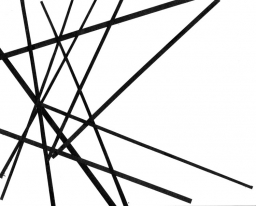Did you find an error or inaccuracy? Feel free to write us. Thank you!Tips to related online calculators
Line slope calculator is helpful for basic calculations in analytic geometry. The coordinates of two points in the plane calculate slope, normal and parametric line equation(s), slope, directional angle, direction vector, the length of the segment, intersections of the coordinate axes, etc.
Our vector sum calculator can add two vectors given by their magnitudes and by included angle.

## Related math problems and questions:

• LineLine p passing through A[-10, 6] and has direction vector v=(3, 2). Is point B[7, 30] on the line p?
• Lie/do not lie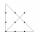The function is given by the rule f(x) = 8x+16. Determine whether point D[-1; 8] lies on this function. Solve graphically or numerically and give reasons for the your answer.
• V - slopeThe slope of the line whose equation is -3x -9 = 0 is
• Line segmentFor the line segment whose endpoints are L[-1, 13] and M[18, 2], find the x and y value for the point located 4 over 7 the distance from L to M.
• Ascent and descent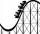Car goes 114 km track, which consists of ascent and descent at time of 1 hour 35 minutes. When climbing moves at the speed 48 km/h and downhill 25 m/s. What is the length of climb and descent?
• Derivation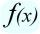Exists a function whose derivation is the same function?
• Slope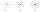Calculate the slope of a line that intersects points (-84,41) and (-76,-32).
• 3d vector componentThe vector u = (3.9, u3) and the length of the vector u is 12. What is is u3?
• Coordinates of vector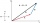Determine the coordinate of a vector u=CD if C(19;-7) and D(-16;-5)
• Curve and lineThe equation of a curve C is y=2x² -8x+9, and the equation of a line L is x+ y=3 (1) Find the x coordinates of the points of intersection of L and C. (2) Show that one of these points is also the stationary point of C?
• Perpendicular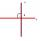Determine the slope of the line perpendicular to the line p: y = -x +4.
• Three points 4The line passed through three points - see table: x y -6 4 -4 3 -2 2 Write line equation in y=mx+b form
• The railway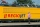The railway line has a gradient of 12 per mille. How many meters will it ascend to a horizontal distance of 4 km?
• VectorsVector a has coordinates (8; 10) and vector b has coordinates (0; 17). If the vector c = b - a, what is the magnitude of the vector c?
• Points on line segmentPoints P & Q belong to segment AB. If AB=a, AP = 2PQ = 2QB, find the distance: between point A and the midpoint of the segment QB.
• Using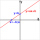Using the point-slope equation, find the equation containing (-7, 3) and slope m = -4
• AngleA straight line p given by the equation y = (-8)/(6) x +78. Calculate the size of angle in degrees between line p and y-axis.